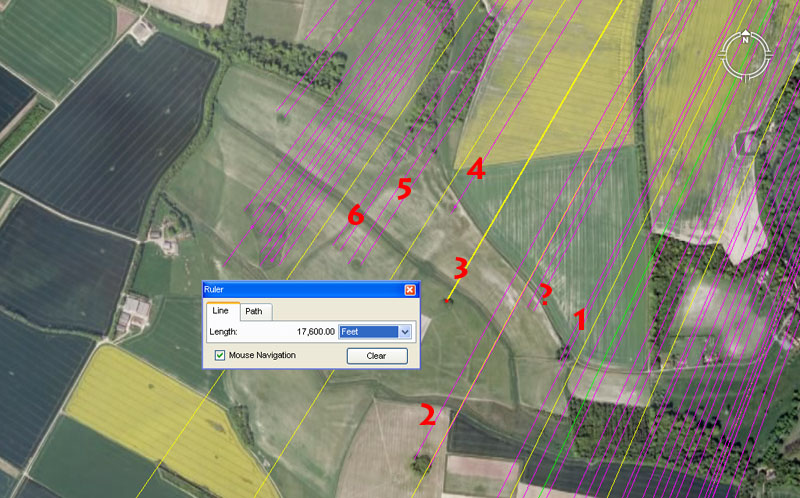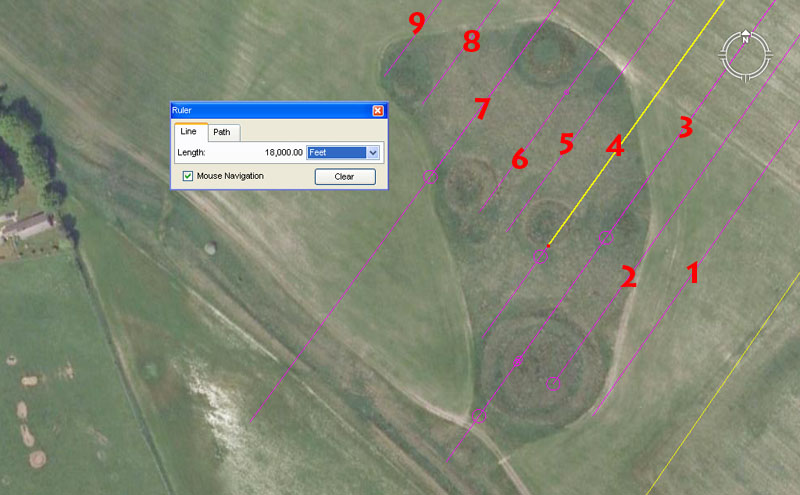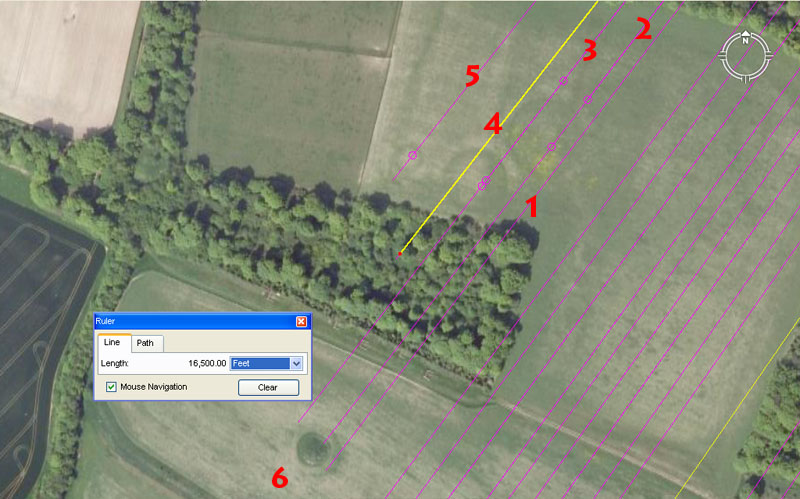NORTH KITE - LAKE DOWNS GROUPSSituated between the Wilsford Barrows and Lake Downs group are these field barrows associated with the North Kite Enclosure. We'll extract a sampling of "distance and angle" codes from this interim group before looking at the more compact set of barrows at Lake Downs.

1. The distance is 17600' and the angle is 26.4-degrees.

This length out from the centre of Durrington Walls Henge is 31/3rd miles. Remember that a mile is also 1760-yards.

The degree angle is also related to the mile of 5280' and the sum of 2640' is half a mile.

? A dark spot in the Google Earth photo probably represents a small mound situated 17280' and at an angle of 27.7777-degrees return to Durrington Walls Henge. There seems to be sufficient scope in the position for another vector, with a return angle of 28-degrees to Durrington Walls.

The sum of 17280' equates to 10,000 of the largest of the Egyptian Royal Cubits @ 1.728' or 20.736". This distance across the Salisbury Plains would be 1/7560th of the equatorial circumference of 24883.2 Greek miles. Remember, the Great Pyramid's intended-design, architectural length was 756' per side.

The angle of 27.777777-degrees would be an expression of 250 ÷ 9. The angle of 28-degrees would set up a mathematical progression used in both calendar and navigational computations.

2. The distance is 19008' and angle is 28.8-degrees.

As stated earlier, the 19008' value is part of the 11.52 mathematical progression related to the equatorial circumference of the Earth. Any of the 3 main systems anciently used for configuring the equatorial circumference in factorable numbers, when rendered in feet and divided by 1900800, will give the value for 1-degree of arc in miles. The sum of 19008' would equate to 3.2 ancient Scottish miles of 5940'.

The degree angle, accentuating the value 288, is one of the most dynamic and useful of antiquity. The Egyptian pyramid acre was 28800 square feet. The Geomancer's mile was 14400' (half of 28800). The value, used in a mathematical progression, produces numbers that be applied to calculations within navigation and the equatorial circumference of the Earth, the Precession of the Equinoxes, lunar and calendar periods.

3. The distance is 17600' and the angle is 30.24-degrees.

The angle is in homage to the value describing 1/2 a degree of equatorial arc. One circumnavigation of the Great Pyramid @ 756' per side = 3024' for a world configured to be 24883.2 Greek miles around the equator. From the same mound on the Salisbury Plains a tutorial related to 30.25-degrees would also have been included to identify 1/2 a degree of arc in a world configured to be 24750 miles of 5280'.

4. The distance is 16800 and the angle is 31.5-degrees.

The value 168 is also very important in mathematical progressions to do with navigation and 16.8' would equate to 1/6th of 1-second of arc under the 24883.2 Greek mile assignment. The value seems to have been popular in 3,4,5 triangles and there was one pressed into service, obviously using ropes, around the Stonehenge site, where the 56 posts of the Aubrey circle provided calibration intervals. That 3.4.5 Triangle had sides that were 126', 168' and 210'.

The degree angle refers to the value 315 and the length of 315' was half a Greek stadia (630'). The inner lintel rim of the Sarsen Circle at Stonehenge has a diameter in one direction of 100.8' (1-second of arc) but 100' @ 90-degrees opposed. This means an inner sweep was meant to convey either 314.16' (in homage to PI or 315' (half a stadia). The value 3.15 was used as a form of PI in certain computations. The value 31.5, used in a mathematical progression generated very valuable numbers for use in navigation or lunar computations. There would be 216 intervals of 31.5-days in the 6804-day lunar nutation cycle. There would be 81 (9 X 9) intervals of 31.5-days in the 2551.5-day period monitored within the Sabbatical Calendar. There would be 11.25 intervals of 31.5-days in the 354.375-day lunar year. Remember, 11.25-degrees equates to 1/32nd of a 360-degree circle and the extended lunar periods could be easily followed in a 360-degree circuit. The sum of 31.5-days = 756-hours. Remember, the Great Pyramid is 756' long.

4b. The distance is 16875' and the angle remains 31.5.

The distance code relates to the a lunar year and related lunar cycles, as well as to navigation. The value sets up an important mathematical progression.

The angle code relates to a rounded form of PI (3.15) and navigation by the Greek mile method. Half a Greek stadia was 315'.

5. The distance is 17718.75000000 and the angle is 33-degrees.

The distance code is in homage to the lunar year (354.375-days) wherein the half period is 177.1875-days or 4252.5-hours. This very important code was built into the angle between the impressive henge ring at Berwick St. James and the centre crown of Barbury Hill, which lies 23-miles to the NNE at an azimuth angle of 17.71875-degrees.

5b. The distance is 17777.7777' and the angle is 33.33333-degrees.

The distance code is simply a progression where each 9th value resolves to an expression of 8, 16, 32, 64, 128, etc. The ancient French Pied du Roi was based upon this sequence and this distance across the Salisbury plains would equate to 16666.66666 ancient French feet (@ 1.0666666' or 12.8").

The angle is 1/3rd of 100.

6. The distance is 17718.75' and the angle is 33.75-degrees.

The angle code sets up a very important mathematical progression that relates to the lunar cycle and navigation simultaneously.

6b. The distance is 17718.75 and the angle is 34.02-degrees.

The angle code relates to the lunar nutation cycle of 6804-days (3402 X 2). The southern circle of Avebury Henge has a diameter of 340.2', as does the Brodgar Ring of Scotland. The Khafre Pyramid @ 708.75' per side is 34020" for one complete circuit.The barrows of Lake Downs. At the bottom right of the picture a yellow line is seen to be bypassing the Lake Downs cluster. The line resolves onto a former ring barrow, now reduced to being a very vivid crop-mark, at 26400' from the centre of Durrington Walls with a return angle of 34.56-degrees. The distance is exactly 5-miles and the return angle relates to the 24883.2-mile circumference, wherein 345.6-miles would equate to 1/72nd of the distance around the equator.

The numbered lines coming in from the centre of Durrington Walls Henge provide the following codes.

1. Like the horns of Isis or Herne, this vector resolves to the side of the barrow orb @ 18150' at an angle of 35-degrees.

The value 18150' is coding in homage to the geodetic system that configures the Earth to 24750 British miles of equatorial circumference. Under this navigational system (based upon the "11" family of numbers), the length of 18150 would be 1/7200th of the equatorial circumference. Similarly, a distance of 18144' (which was dual coded into this position) would represent 1/7200th of the circumference for a world that was 24883.2 Greek miles around ("6&7" number families combined).

The degree angle sets up a "7" progression, very useful for navigational or calendar calculations.

2. The distance is 18144' and the angle is 35.2-degrees.

The angle code provides a mathematical progression that relates to the British standard mile and the sum of 352' would be 1/15th of a mile.

3. This line carries five length codes, but one overall angle code. The first length is 17920' and the angle is 35.4375-degrees.

The 17920' coding (56 X320) is based upon a "7" number progression (7, 14, 28, 56) that would have been useful in calendar counts within a circuit of 320 segmentations. The Aubrey Circle at Stonehenge had 56 posts for such counts (lunar in particular). The value also has applications in navigation and the sum of 17920' would be 1/7290th of the equatorial circumference of the Earth under the 24883.2-Greek mile reading. Note: The sum of 7.29" was 1/8th of a Roman pace (58.32") and represented part of a dynamic lunar mathematical progression. The Bush Barrow Lozenge artefact found at Normanton Downs measured 7.29", point to point in its longest length.

The angle relates to the duration of the lunar year @ 354.375-days.

3b. the length is 18144' and the angle remains 35.4375-degrees.

3c. The length is 18150' and the angle remains 35.4375-degrees.

The length would be 1/7200th of the equatorial circumference of the Earth under the 24750-mile navigational system ("11" family of numbers).

3d. The length is 18265' and the angle remains 35.4375-degrees.

The sum of 182.65-days equates to half a solar year or the number of days between equinoxes.

3e. The length is 18333.333333' and the angle remains 35.4375-degrees.

The sum of 18333.333333' would be an expression of the "11" family of increments, including the fathom, rod, chain, furlong, mile and league. The sum of 3 X 18333.3333' would be 55000' (10000 ancient fathoms).

4. The distance is 18000 and the angle is 35.64-degrees.

A distance of 18000' would equate to 1/7260th of the equatorial circumference of the Earth under the 24750-mile assignment. The 7260 value has great significance in a mathematical progression related to the 130680000' (24750-mile) navigational method.

The angle code is also navigational. The "Y" Holes circle at Stonehenge was configured to have a diameter of 178.2' (half of 356.4'). This could be read as either 103.68 of the "11" family Royal Cubits of 20.625" each (coding the rate at which the Earth rotates per hour ... 1036.8 MPH ...24883.2 ÷ 24). It could also be read as 103.125* of the largest Egyptian Royal Cubits @ 20.736" each. Alternatively it could be read as 86.4 increments of 24.75".

*Footnote: The double value of 103.125 is 206.25

The equatorial circumference of 24750-miles could be broken down into 11th parts within a 360-degree circle, such that 22.5-degrees of arc equated to 1100 miles. Alternatively, the 24750-mile circumference, rendered in feet (130680000'), could be broken down into, say, 1/440000th parts, or 2940' (equating to 35640" or 540 fathoms) etc. The Earth's equatorial circumference, under the "11" family of numbers, was still broken down into 4ths, 8ths, 16ths, 32nds, 64ths, etc., in a 360-degree circuit and the lesser divisions read as "11" family of numbers values. It was necessary to do so for positional plotting at sea.

It's interesting, mathematically, to note that the value in inches of the "11" series Royal Cubit, when multiplied by the "foot" value of the largest Egyptian Royal Cubit (1.728' or 20.736") = 35.64. This meant that the 24750-mile (or 130680000') circumference equated to 75625000 of the largest Egyptian Royal Cubits @ 20.736" each. The sum of 756.25' equated to 1/8th of 1-minute of equatorial arc (6025') under the Great Pyramid's second navigational method, which symbolically recognised that the length of the edifice was 756.25' rather than the literal value of 756' (where 1-minute of arc was 6048' .... for a world that was 24883.2 Greek miles in circumference ..."6&7" family of numbers).

This somewhat convoluted exercise shows the degree of manipulation of numbers going on in the tutorials presented to initiate students, who had to memorise how all of the various factorable values interacted with each other.

4b. The length is 18150' and the angle remains 35.64'.

5. The length is 18000' and the angle is 35.84-degrees.

The value 35.84 (3521/25ths acts as a catalyst or ratio to convert the 12 X 12 X 12 X 12 X 12 X 1.2 - Greek-mile equatorial circumference of the Earth (130636800') into lunar and compass related numbers simultaneously. One can, therefore, divide both 1-minute (6048') or 1-second of arc (100.8') by this value to create a lunar-compass progression.

6. The distance is 17777.77777' and the angle is 36-degrees.

The distance value is dual coded and refers to dividing 160000' by 9. Alternatively, and more importantly, a second reading of 17718.75 would have been encoded into the northwestern-most edge of this mound (17718.75 X 2 = 35437.5) as the lunar year is 354.375-days, giving a half-period of 177.1875-days.

The degree angle, of course, simply relates to the 360-degree compass.

6b. The distance is 18000' and the angle remains 36-degrees.

Both distance and angle are self explanatory.

7. The distance remains 18000' and the angle shifts marginally to 36.288-degrees.

In the 24883.2 Greek mile equatorial circumference, 1-degree of arc is 362880' and 1-minute of arc is 36288".

7b. The distance is 18457.03125' and the angle remains 36.288-degrees.

The distance can be dual coded to express an interval within the lunar month of 29.53125-days of which 1.845703125-days (1433/512nds) would be a 1/16th part. Likewise, The Precession of the Equinoxes endure for 25920-years and the half period is 12960-years. This distance out from Durrington Walls Henge could carry the tutorial of 18514.2857143' (185142/7ths-days) and relate to 1/14th part of the Precessional cycle (1841.42857143-days). This school was not for the faint-hearted, but was reserved for over-achievers. There would certainly have been days when confused or overwhelmed students complained, "Today I'm all sixes and sevens".

8. The distance is 17920' and the angle is 36.525-degrees.

The distance code is an expression of the conversion ratio 3584 (mentioned earlier) and 1792 is the half value of that number.

The angle simply relates to the solar year of 365.25-days.

9. The distance remains 17920' and the angle swings to 36.9140625-degrees ( 36117/128ths).

This angle to the western outer limits of the mound complex is a fraction expression that relates to the lunar month value. The angle is 1/8th of 295.3125.These are some of the ring mounds or former barrows (now reduced to being crop marks) that sit just northwest of the Lake Barrows group. Here are the apparent codes of position that were being taught to ancient students of navigation:

1. If one of the horns of Herne or Isis was meant to sweep the eastern edge of the mound, then the distance appears to be 17066.66666', at an angle is 37.33333-degrees.

The length is an expression of 51200' (1/3rd) and a progression based on 512 creates a string of very useful lunar or navigational values.

The angle is (1/3rd) of 112-degrees and the values 112, 224, etc., formed a progression used in traditional English weights standards (CWT, Ton, etc.), which had precedents with the Egyptian Deben weight standard.

2. Has at least 3 codes of length and one of angle associated with it, the first of which is: 15840' at a return angle back to Durrington Walls Henge of 37.5-degrees.

The distance of 15840' would equate to exactly 3 British miles.

The angle of 37.5-degrees provides a dynamic value that is very useful in lunar and 360-degree compass calculations.

2b. The distance is 16000' and the angle remains 37.5-degrees.

The sum of 16000' is self explanatory.

2c. The distance is 17010' and the angle remains 37.5-degrees.

The distance code relates to the 6804-day lunar nutation cycle, of which 1701-days would be 1/4th of the cycle. Remember, a dual mound marker, giving a perfect due East reading from the centre of Durrington Walls Henge sits 17010' out.

3. This line has four length codes and one angle code, the first of which 15840' (3-miles) at an angle of 37.8-degrees.

The angle code is navigational and 1/2 of the length of the Great Pyramid is 378' or 1/16th of 1-minute of equatorial arc (6048').

3b. The distance is 16180.4' and the angle remains 37.8-degrees.

The distance relates to the PHI ratio (1.618034 to 1). Remember: another marker sits at this distance from Durrington Walls Henge at an azimuth angle of 83.3333-degrees.

3c. The distance is 16200' and the angle remains 37.8-degrees.

Where ever true PHI is represented, it also infers "rounded PHI @ 1.62 ... and in this case at the larger value of 16200'.

3d. The distance is 17010' (lunar nutation cycle) and the angle remains 37.8-degrees.

4. This band of forest sits exactly one league (16500') from the centre of Durrington walls and a circle aspect within the trees might indicate a mound at 38.016-degrees return.

The degree angle relates to navigation and each of the equatorial circumference values within the 3-main navigational systems, rendered in feet, will produce the value for half a degree of arc (in miles) when divided by 3801600.

5. A small crop-mark mound seems to carry codes front and rear, the first of which 16250' at an angle of 38.5-degrees.

The distance code would relate to a progression used in Calendar calculations, with a year composed of 13-months of 28-days each (364-days). The sum of 1.625 = 1/8th of 13.

The degree angle of 38.5 is half of 77.

5b. The distance is 16335' and the angle remains 38.5-degrees.

The distance would equate to 1/8000th of the equatorial circumference under the 24750-mile assignment.

Continue